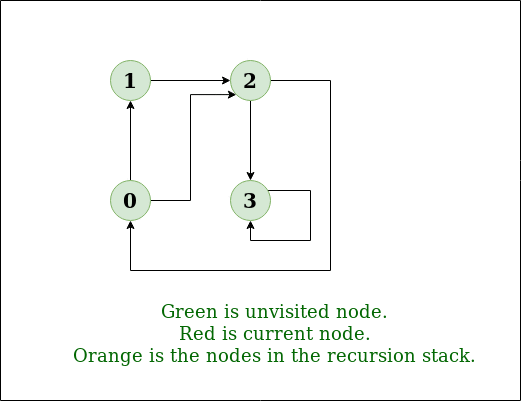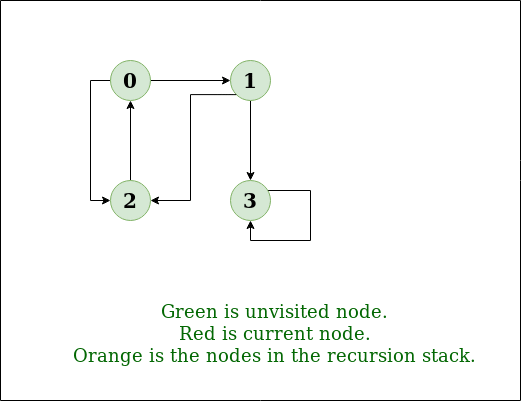# Depth First Search or DFS for a Graph

• Difficulty Level : Easy
• Last Updated : 30 May, 2022

Depth First Traversal (or Search) for a graph is similar to Depth First Traversal of a tree. The only catch here is, unlike trees, graphs may contain cycles (a node may be visited twice). To avoid processing a node more than once, use a boolean visited array.

Example:

Input: n = 4, e = 6
0 -> 1, 0 -> 2, 1 -> 2, 2 -> 0, 2 -> 3, 3 -> 3
Output: DFS from vertex 1 : 1 2 0 3
Explanation:
DFS Diagram:Input: n = 4, e = 6
2 -> 0, 0 -> 2, 1 -> 2, 0 -> 1, 3 -> 3, 1 -> 3
Output: DFS from vertex 2 : 2 0 1 3
Explanation:
DFS Diagram:Prerequisites:
See this post for all applications of Depth First Traversal.

Approach:
Depth-first search is an algorithm for traversing or searching tree or graph data structures. The algorithm starts at the root node (selecting some arbitrary node as the root node in the case of a graph) and explores as far as possible along each branch before backtracking. So the basic idea is to start from the root or any arbitrary node and mark the node and move to the adjacent unmarked node and continue this loop until there is no unmarked adjacent node. Then backtrack and check for other unmarked nodes and traverse them. Finally, print the nodes in the path.

Algorithm:
Create a recursive function that takes the index of the node and a visited array.

1. Mark the current node as visited and print the node.
2. Traverse all the adjacent and unmarked nodes and call the recursive function with the index of the adjacent node.

Implementation:
Below are implementations of simple Depth First Traversal. The C++ implementation uses an adjacency list representation of graphs. STL’s list container is used to store lists of adjacent nodes.

## C++

 `// C++ program to print DFS traversal from``// a given vertex in a  given graph``#include ``using` `namespace` `std;` `// Graph class represents a directed graph``// using adjacency list representation``class` `Graph {``public``:``    ``map<``int``, ``bool``> visited;``    ``map<``int``, list<``int``> > adj;` `    ``// function to add an edge to graph``    ``void` `addEdge(``int` `v, ``int` `w);` `    ``// DFS traversal of the vertices``    ``// reachable from v``    ``void` `DFS(``int` `v);``};` `void` `Graph::addEdge(``int` `v, ``int` `w)``{``    ``adj[v].push_back(w); ``// Add w to v’s list.``}` `void` `Graph::DFS(``int` `v)``{``    ``// Mark the current node as visited and``    ``// print it``    ``visited[v] = ``true``;``    ``cout << v << ``" "``;` `    ``// Recur for all the vertices adjacent``    ``// to this vertex``    ``list<``int``>::iterator i;``    ``for` `(i = adj[v].begin(); i != adj[v].end(); ++i)``        ``if` `(!visited[*i])``            ``DFS(*i);``}` `// Driver code``int` `main()``{``    ``// Create a graph given in the above diagram``    ``Graph g;``    ``g.addEdge(0, 1);``    ``g.addEdge(0, 2);``    ``g.addEdge(1, 2);``    ``g.addEdge(2, 0);``    ``g.addEdge(2, 3);``    ``g.addEdge(3, 3);` `    ``cout << ``"Following is Depth First Traversal"``            ``" (starting from vertex 2) \n"``;``    ``g.DFS(2);` `    ``return` `0;``}` `// improved by Vishnudev C`

## Java

 `// Java program to print DFS``// traversal from a given``// graph``import` `java.io.*;``import` `java.util.*;` `// This class represents a``// directed graph using adjacency``// list representation``class` `Graph {``    ``private` `int` `V; ``// No. of vertices` `    ``// Array  of lists for``    ``// Adjacency List Representation``    ``private` `LinkedList adj[];` `    ``// Constructor``    ``@SuppressWarnings``(``"unchecked"``) Graph(``int` `v)``    ``{``        ``V = v;``        ``adj = ``new` `LinkedList[v];``        ``for` `(``int` `i = ``0``; i < v; ++i)``            ``adj[i] = ``new` `LinkedList();``    ``}` `    ``// Function to add an edge into the graph``    ``void` `addEdge(``int` `v, ``int` `w)``    ``{``        ``adj[v].add(w); ``// Add w to v's list.``    ``}` `    ``// A function used by DFS``    ``void` `DFSUtil(``int` `v, ``boolean` `visited[])``    ``{``        ``// Mark the current node as visited and print it``        ``visited[v] = ``true``;``        ``System.out.print(v + ``" "``);` `        ``// Recur for all the vertices adjacent to this``        ``// vertex``        ``Iterator i = adj[v].listIterator();``        ``while` `(i.hasNext()) {``            ``int` `n = i.next();``            ``if` `(!visited[n])``                ``DFSUtil(n, visited);``        ``}``    ``}` `    ``// The function to do DFS traversal.``    ``// It uses recursive``    ``// DFSUtil()``    ``void` `DFS(``int` `v)``    ``{``        ``// Mark all the vertices as``        ``// not visited(set as``        ``// false by default in java)``        ``boolean` `visited[] = ``new` `boolean``[V];` `        ``// Call the recursive helper``        ``// function to print DFS``        ``// traversal``        ``DFSUtil(v, visited);``    ``}` `    ``// Driver Code``    ``public` `static` `void` `main(String args[])``    ``{``        ``Graph g = ``new` `Graph(``4``);` `        ``g.addEdge(``0``, ``1``);``        ``g.addEdge(``0``, ``2``);``        ``g.addEdge(``1``, ``2``);``        ``g.addEdge(``2``, ``0``);``        ``g.addEdge(``2``, ``3``);``        ``g.addEdge(``3``, ``3``);` `        ``System.out.println(``            ``"Following is Depth First Traversal "``            ``+ ``"(starting from vertex 2)"``);` `        ``g.DFS(``2``);``    ``}``}``// This code is contributed by Aakash Hasija`

## Python3

 `# Python3 program to print DFS traversal``# from a given  graph``from` `collections ``import` `defaultdict` `# This class represents a directed graph using``# adjacency list representation`  `class` `Graph:` `    ``# Constructor``    ``def` `__init__(``self``):` `        ``# default dictionary to store graph``        ``self``.graph ``=` `defaultdict(``list``)` `    ``# function to add an edge to graph``    ``def` `addEdge(``self``, u, v):``        ``self``.graph[u].append(v)` `    ``# A function used by DFS``    ``def` `DFSUtil(``self``, v, visited):` `        ``# Mark the current node as visited``        ``# and print it``        ``visited.add(v)``        ``print``(v, end``=``' '``)` `        ``# Recur for all the vertices``        ``# adjacent to this vertex``        ``for` `neighbour ``in` `self``.graph[v]:``            ``if` `neighbour ``not` `in` `visited:``                ``self``.DFSUtil(neighbour, visited)` `    ``# The function to do DFS traversal. It uses``    ``# recursive DFSUtil()``    ``def` `DFS(``self``, v):` `        ``# Create a set to store visited vertices``        ``visited ``=` `set``()` `        ``# Call the recursive helper function``        ``# to print DFS traversal``        ``self``.DFSUtil(v, visited)` `# Driver code`  `# Create a graph given``# in the above diagram``g ``=` `Graph()``g.addEdge(``0``, ``1``)``g.addEdge(``0``, ``2``)``g.addEdge(``1``, ``2``)``g.addEdge(``2``, ``0``)``g.addEdge(``2``, ``3``)``g.addEdge(``3``, ``3``)` `print``(``"Following is DFS from (starting from vertex 2)"``)``g.DFS(``2``)` `# This code is contributed by Neelam Yadav`

## C#

 `// C# program to print DFS traversal``// from a given graph``using` `System;``using` `System.Collections.Generic;` `// This class represents a directed graph``// using adjacency list representation``class` `Graph {``    ``private` `int` `V; ``// No. of vertices` `    ``// Array of lists for``    ``// Adjacency List Representation``    ``private` `List<``int``>[] adj;` `    ``// Constructor``    ``Graph(``int` `v)``    ``{``        ``V = v;``        ``adj = ``new` `List<``int``>[ v ];``        ``for` `(``int` `i = 0; i < v; ++i)``            ``adj[i] = ``new` `List<``int``>();``    ``}` `    ``// Function to Add an edge into the graph``    ``void` `AddEdge(``int` `v, ``int` `w)``    ``{``        ``adj[v].Add(w); ``// Add w to v's list.``    ``}` `    ``// A function used by DFS``    ``void` `DFSUtil(``int` `v, ``bool``[] visited)``    ``{``        ``// Mark the current node as visited``        ``// and print it``        ``visited[v] = ``true``;``        ``Console.Write(v + ``" "``);` `        ``// Recur for all the vertices``        ``// adjacent to this vertex``        ``List<``int``> vList = adj[v];``        ``foreach``(``var` `n ``in` `vList)``        ``{``            ``if` `(!visited[n])``                ``DFSUtil(n, visited);``        ``}``    ``}` `    ``// The function to do DFS traversal.``    ``// It uses recursive DFSUtil()``    ``void` `DFS(``int` `v)``    ``{``        ``// Mark all the vertices as not visited``        ``// (set as false by default in c#)``        ``bool``[] visited = ``new` `bool``[V];` `        ``// Call the recursive helper function``        ``// to print DFS traversal``        ``DFSUtil(v, visited);``    ``}` `    ``// Driver Code``    ``public` `static` `void` `Main(String[] args)``    ``{``        ``Graph g = ``new` `Graph(4);` `        ``g.AddEdge(0, 1);``        ``g.AddEdge(0, 2);``        ``g.AddEdge(1, 2);``        ``g.AddEdge(2, 0);``        ``g.AddEdge(2, 3);``        ``g.AddEdge(3, 3);` `        ``Console.WriteLine(``            ``"Following is Depth First Traversal "``            ``+ ``"(starting from vertex 2)"``);` `        ``g.DFS(2);``        ``Console.ReadKey();``    ``}``}` `// This code is contributed by techno2mahi`

## Javascript

 ``

Output:

```Following is Depth First Traversal (starting from vertex 2)
2 0 1 3```

Complexity Analysis:

• Time complexity: O(V + E), where V is the number of vertices and E is the number of edges in the graph.
• Space Complexity: O(V), since an extra visited array of size V is required.

Handling A Disconnected Graph:

• Solution:
This will happen by handling a corner case.
The above code traverses only the vertices reachable from a given source vertex. All the vertices may not be reachable from a given vertex, as in a Disconnected graph. To do a complete DFS traversal of such graphs, run DFS from all unvisited nodes after a DFS.
The recursive function remains the same.
• Algorithm:
1. Create a recursive function that takes the index of the node and a visited array.
2. Mark the current node as visited and print the node.
3. Traverse all the adjacent and unmarked nodes and call the recursive function with the index of the adjacent node.
4. Run a loop from 0 to the number of vertices and check if the node is unvisited in the previous DFS, call the recursive function with the current node.

Implementation:

## C++

 `// C++ program to print DFS``// traversal for a given given``// graph``#include ``using` `namespace` `std;` `class` `Graph {` `    ``// A function used by DFS``    ``void` `DFSUtil(``int` `v);` `public``:``    ``map<``int``, ``bool``> visited;``    ``map<``int``, list<``int``> > adj;``    ``// function to add an edge to graph``    ``void` `addEdge(``int` `v, ``int` `w);` `    ``// prints DFS traversal of the complete graph``    ``void` `DFS();``};` `void` `Graph::addEdge(``int` `v, ``int` `w)``{``    ``adj[v].push_back(w); ``// Add w to v’s list.``}` `void` `Graph::DFSUtil(``int` `v)``{``    ``// Mark the current node as visited and print it``    ``visited[v] = ``true``;``    ``cout << v << ``" "``;` `    ``// Recur for all the vertices adjacent to this vertex``    ``list<``int``>::iterator i;``    ``for` `(i = adj[v].begin(); i != adj[v].end(); ++i)``        ``if` `(!visited[*i])``            ``DFSUtil(*i);``}` `// The function to do DFS traversal. It uses recursive``// DFSUtil()``void` `Graph::DFS()``{``    ``// Call the recursive helper function to print DFS``    ``// traversal starting from all vertices one by one``    ``for` `(``auto` `i : adj)``        ``if` `(visited[i.first] == ``false``)``            ``DFSUtil(i.first);``}` `// Driver  Code``int` `main()``{``    ``// Create a graph given in the above diagram``    ``Graph g;``    ``g.addEdge(0, 1);``    ``g.addEdge(0, 9);``    ``g.addEdge(1, 2);``    ``g.addEdge(2, 0);``    ``g.addEdge(2, 3);``    ``g.addEdge(9, 3);` `    ``cout << ``"Following is Depth First Traversal \n"``;``    ``g.DFS();` `    ``return` `0;``}``// improved by Vishnudev C`

## Java

 `// Java program to print DFS``// traversal from a given given``// graph``import` `java.io.*;``import` `java.util.*;` `// This class represents a``// directed graph using adjacency``// list representation``class` `Graph {``    ``private` `int` `V; ``// No. of vertices` `    ``// Array  of lists for``    ``// Adjacency List Representation``    ``private` `LinkedList adj[];` `    ``// Constructor``    ``@SuppressWarnings``(``"unchecked"``) Graph(``int` `v)``    ``{``        ``V = v;``        ``adj = ``new` `LinkedList[v];``        ``for` `(``int` `i = ``0``; i < v; ++i)``            ``adj[i] = ``new` `LinkedList();``    ``}` `    ``// Function to add an edge into the graph``    ``void` `addEdge(``int` `v, ``int` `w)``    ``{``        ``adj[v].add(w); ``// Add w to v's list.``    ``}` `    ``// A function used by DFS``    ``void` `DFSUtil(``int` `v, ``boolean` `visited[])``    ``{``        ``// Mark the current node as visited and print it``        ``visited[v] = ``true``;``        ``System.out.print(v + ``" "``);` `        ``// Recur for all the vertices adjacent to this``        ``// vertex``        ``Iterator i = adj[v].listIterator();``        ``while` `(i.hasNext()) {``            ``int` `n = i.next();``            ``if` `(!visited[n])``                ``DFSUtil(n, visited);``        ``}``    ``}` `    ``// The function to do DFS traversal. It uses recursive``    ``// DFSUtil()``    ``void` `DFS()``    ``{``        ``// Mark all the vertices as not visited(set as``        ``// false by default in java)``        ``boolean` `visited[] = ``new` `boolean``[V];` `        ``// Call the recursive helper function to print DFS``        ``// traversal starting from all vertices one by one``        ``for` `(``int` `i = ``0``; i < V; ++i)``            ``if` `(visited[i] == ``false``)``                ``DFSUtil(i, visited);``    ``}` `    ``// Driver Code``    ``public` `static` `void` `main(String args[])``    ``{``        ``Graph g = ``new` `Graph(``4``);` `        ``g.addEdge(``0``, ``1``);``        ``g.addEdge(``0``, ``2``);``        ``g.addEdge(``1``, ``2``);``        ``g.addEdge(``2``, ``0``);``        ``g.addEdge(``2``, ``3``);``        ``g.addEdge(``3``, ``3``);` `        ``System.out.println(``            ``"Following is Depth First Traversal"``);` `        ``g.DFS();``    ``}``}``// This code is contributed by Aakash Hasija`

## Python3

 `'''Python program to print DFS traversal for complete graph'''``from` `collections ``import` `defaultdict` `# this class represents a directed graph using adjacency list representation`  `class` `Graph:``    ``# Constructor``    ``def` `__init__(``self``):``        ``# default dictionary to store graph``        ``self``.graph ``=` `defaultdict(``list``)` `    ``# Function to add an edge to graph``    ``def` `addEdge(``self``, u, v):``        ``self``.graph[u].append(v)``    ``# A function used by DFS` `    ``def` `DFSUtil(``self``, v, visited):``        ``# Mark the current node as visited and print it``        ``visited.add(v)``        ``print``(v,end``=``" "``)` `        ``# recur for all the vertices adjacent to this vertex``        ``for` `neighbour ``in` `self``.graph[v]:``            ``if` `neighbour ``not` `in` `visited:``                ``self``.DFSUtil(neighbour, visited)``        ``# The function to do DFS traversal. It uses recursive DFSUtil` `    ``def` `DFS(``self``):``        ``# create a set to store all visited vertices``        ``visited ``=` `set``()``        ``# call the recursive helper function to print DFS traversal starting from all``        ``# vertices one by one``        ``for` `vertex ``in` `self``.graph:``            ``if` `vertex ``not` `in` `visited:``                ``self``.DFSUtil(vertex, visited)``# Driver code``# create a graph given in the above diagram` `print``(``"Following is Depth First Traversal \n"``)``g ``=` `Graph()``g.addEdge(``0``, ``1``)``g.addEdge(``0``, ``2``)``g.addEdge(``1``, ``2``)``g.addEdge(``2``, ``0``)``g.addEdge(``2``, ``3``)``g.addEdge(``3``, ``3``)``g.DFS()` `# Improved by Dheeraj Kumar`

## C#

 `// C# program to print DFS``// traversal from a given given``// graph``using` `System;``using` `System.Collections.Generic;` `// This class represents a``// directed graph using adjacency``// list representation``public` `class` `Graph {``    ``private` `int` `V; ``// No. of vertices` `    ``// Array of lists for``    ``// Adjacency List Representation``    ``private` `List<``int``>[] adj;` `    ``// Constructor``    ``Graph(``int` `v)``    ``{``        ``V = v;``        ``adj = ``new` `List<``int``>[ v ];``        ``for` `(``int` `i = 0; i < v; ++i)``            ``adj[i] = ``new` `List<``int``>();``    ``}` `    ``// Function to add an edge into the graph``    ``void` `addEdge(``int` `v, ``int` `w)``    ``{``        ``adj[v].Add(w); ``// Add w to v's list.``    ``}` `    ``// A function used by DFS``    ``void` `DFSUtil(``int` `v, ``bool``[] visited)``    ``{``        ``// Mark the current``        ``// node as visited and print it``        ``visited[v] = ``true``;``        ``Console.Write(v + ``" "``);` `        ``// Recur for all the``        ``// vertices adjacent to this``        ``// vertex``        ``foreach``(``int` `i ``in` `adj[v])``        ``{``            ``int` `n = i;``            ``if` `(!visited[n])``                ``DFSUtil(n, visited);``        ``}``    ``}` `    ``// The function to do``    ``// DFS traversal. It uses recursive``    ``// DFSUtil()``    ``void` `DFS()``    ``{``        ``// Mark all the vertices as not visited(set as``        ``// false by default in java)``        ``bool``[] visited = ``new` `bool``[V];` `        ``// Call the recursive helper``        ``// function to print DFS``        ``// traversal starting from``        ``// all vertices one by one``        ``for` `(``int` `i = 0; i < V; ++i)``            ``if` `(visited[i] == ``false``)``                ``DFSUtil(i, visited);``    ``}` `    ``// Driver code``    ``public` `static` `void` `Main(String[] args)``    ``{``        ``Graph g = ``new` `Graph(4);` `        ``g.addEdge(0, 1);``        ``g.addEdge(0, 2);``        ``g.addEdge(1, 2);``        ``g.addEdge(2, 0);``        ``g.addEdge(2, 3);``        ``g.addEdge(3, 3);` `        ``Console.WriteLine(``            ``"Following is Depth First Traversal"``);` `        ``g.DFS();``    ``}``}` `// This code is contributed by PrinciRaj1992`

## Javascript

 ``

Output:

```Following is Depth First Traversal
0 1 2 3 9```

Complexity Analysis:

• Time complexity: O(V + E), where V is the number of vertices and E is the number of edges in the graph.
• Space Complexity: O(V), since an extra visited array of size V is required.

https://youtu.be/Y40bRyPQQr0